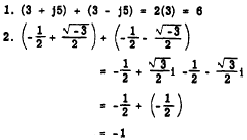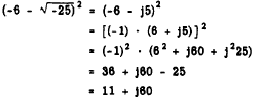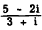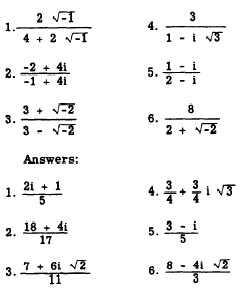Custom SearchCONJUGATES AND SPECIAL PRODUCTS Two complex numbers that are alike except for the sign of their imaginary parts are called CONJUGATE COMPLEX NUMBERS. For example, 3 + 5i and 3 - 5i are conjugates. Either number is the conjugate of the other. If one complex number is known, the conjugate can be obtained immediately by changing the sign of the imaginary part. The conjugate of -8 +is -8 -The conjugate of  -isThe sum of two conjugate complex numbers is a real number, as illustrated by the following:Product of Two Conjugates The product of two conjugate complex numbers is a real number. Multiplying two conjugates is equivalent to finding the product of the sum and difference of two numbers. Consider the following examples:Squaring a Complex Number Squaring a complex number is equivalent to raising a binomial to the second power. For example :DIVISION OF COMPLEX NUMBER When dividing by a pure imaginary, the denomfnator may be rationalized and the problem thus simplified by multiplying both numerator and denominator by the denominator. Thus,Division of complex numbers can be accomplished by multiplying the numerator and denominator by the number that is the conjugate of the denominator. This process is similar to the process of rationalizing a denominator in the case of real numbers that are irrational. As an example, considerThe denominator is 3 + i. Its conjugate is 3 - i. Multiplying numerator and denominator by 3- i givesPractice problems. Rationalize the denominators and simplify: